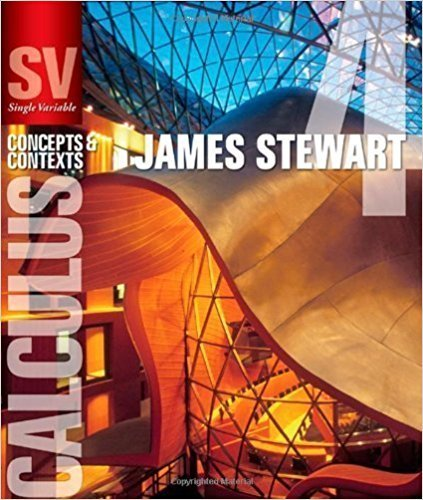×
×

# Solutions for Chapter 5.9: APPROXIMATE INTEGRATION## Full solutions for Single Variable Calculus: Concepts and Contexts (Stewart's Calculus Series) | 4th Edition

ISBN: 9780495559726Solutions for Chapter 5.9: APPROXIMATE INTEGRATION

Solutions for Chapter 5.9
4 5 0 407 Reviews
13
2
##### ISBN: 9780495559726

This expansive textbook survival guide covers the following chapters and their solutions. This textbook survival guide was created for the textbook: Single Variable Calculus: Concepts and Contexts (Stewart's Calculus Series), edition: 4. Since 42 problems in chapter 5.9: APPROXIMATE INTEGRATION have been answered, more than 20099 students have viewed full step-by-step solutions from this chapter. Chapter 5.9: APPROXIMATE INTEGRATION includes 42 full step-by-step solutions. Single Variable Calculus: Concepts and Contexts (Stewart's Calculus Series) was written by and is associated to the ISBN: 9780495559726.

Key Calculus Terms and definitions covered in this textbook
• Annual percentage rate (APR)

The annual interest rate

• Average velocity

The change in position divided by the change in time.

• Categorical variable

In statistics, a nonnumerical variable such as gender or hair color. Numerical variables like zip codes, in which the numbers have no quantitative significance, are also considered to be categorical.

• Center

The central point in a circle, ellipse, hyperbola, or sphere

• Convenience sample

A sample that sacrifices randomness for convenience

• Cube root

nth root, where n = 3 (see Principal nth root),

• Half-plane

The graph of the linear inequality y ? ax + b, y > ax + b y ? ax + b, or y < ax + b.

• Horizontal line

y = b.

• Jump discontinuity at x a

limx:a - ƒ1x2 and limx:a + ƒ1x2 exist but are not equal

• Logarithmic regression

See Natural logarithmic regression

• One-to-one rule of logarithms

x = y if and only if logb x = logb y.

• Principle of mathematical induction

A principle related to mathematical induction.

• Random variable

A function that assigns real-number values to the outcomes in a sample space.

• Rose curve

A graph of a polar equation or r = a cos nu.

• Variable (in statistics)

A characteristic of individuals that is being identified or measured.

• Velocity

A vector that specifies the motion of an object in terms of its speed and direction.

• Vertex form for a quadratic function

ƒ(x) = a(x - h)2 + k

• x-intercept

A point that lies on both the graph and the x-axis,.

• Ymax

The y-value of the top of the viewing window.

• Zero factorial

See n factorial.

×